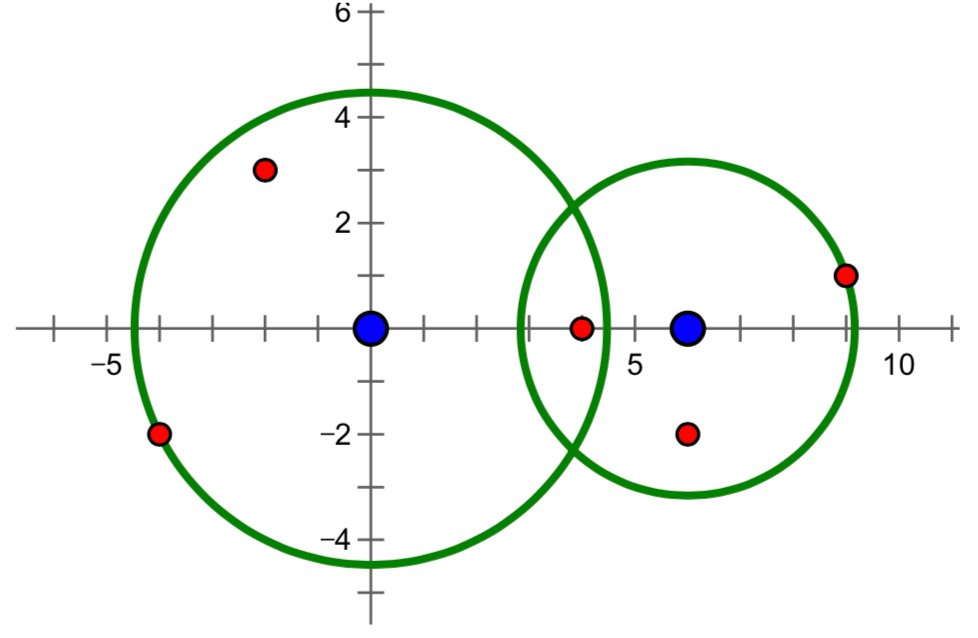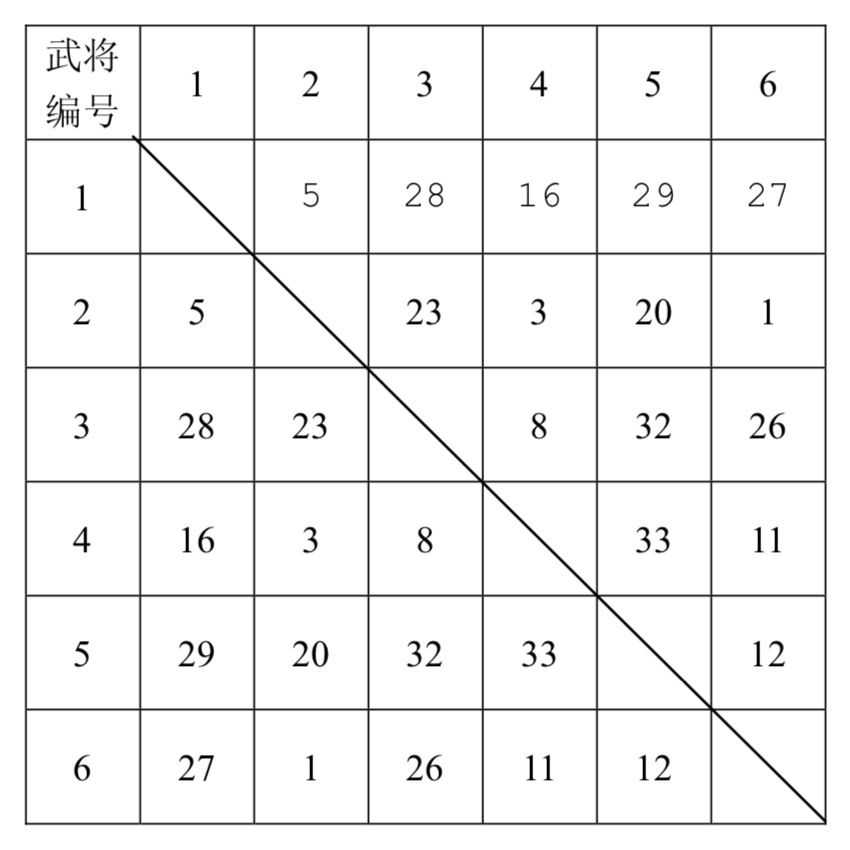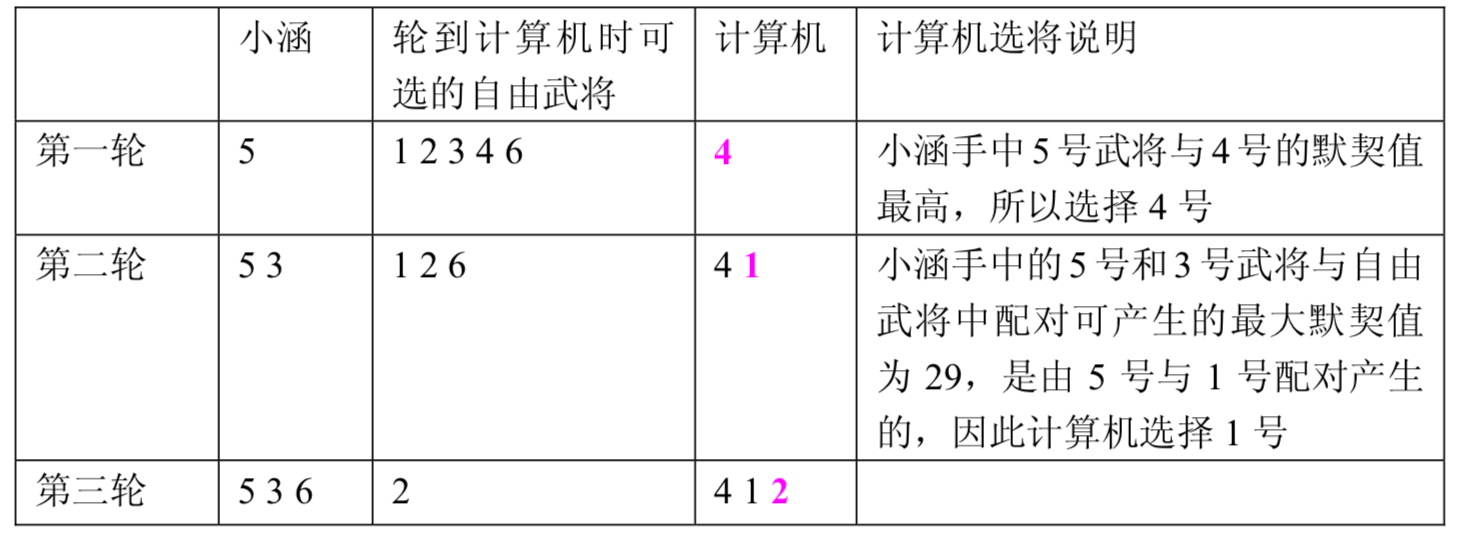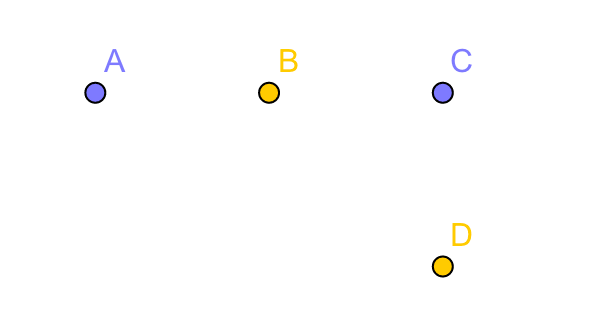NOIP2010 普及组Actinoi 7月 29, 2019

# T1:数字统计

## 输入输出样例

### 输入样例 #1

2 22


### 输出样例 #1

6


### 输入样例 #2

2 100


### 输出样例 #2

20


## 说明

NOIP 2010 普及组 第一题

# 题解：

​ 本题直接模拟就可以。枚举 区间的每一个数字。然后不断地将这个数字 取最后一位判断是不是 ，然后再将这个数字 ，不断循环这两个过程，直到这个数字小于等于 时结束。

#include <iostream>
using namespace std;
int main() {
int l, r;
cin >> l >> r;
int ans = 0; //存储数字2出现的次数
for (int i = l; i <= r; i++) {
int n = i;
while (n > 0) {
if (n % 10 == 2)
ans++;
n /= 10;
}
}
cout << ans << endl;
return 0;
}


# T2:接水问题

## 输入输出样例

### 输入样例 #1

5 3
4 4 1 2 1


### 输出样例 #1

4


### 输入样例 #2

8 4
23 71 87 32 70 93 80 76


### 输出样例 #2

163


## 说明

【输入输出样例 1 说明】

【数据范围】

NOIP 2010 普及组 第二题

# 题解：

​ 本题运用模拟就可以解决。我们设置一个 数组来存储每个水龙头还需要几秒才可以接完，然后一直循环，直到所有人都接上水之后输出最终答案。

#include <iostream>
using namespace std;
int people, tap;
int main() {
int n, m;
cin >> n >> m; //表示接水人数和龙头个数
for (int i = 1; i <= n; i++)
cin >> people[i];
int ans = 0;
int k = 0;
while (1) {
ans++;
for (int i = 1; i <= m; i++) {
if (tap[i] == 0 && k < n) {
k++;
tap[i] = people[k];
}
tap[i]--;
}
if (k >= n) {
int maxx = 0;
for (int i = 1; i <= m; i++)
if (tap[i] > maxx)
maxx = tap[i];
cout << ans + maxx << endl;
return 0;
}
}
return 0;
}


# T3:导弹拦截

## 输入输出样例

### 输入 #1

0 0 10 0
2
-3 3
10 0


### 输出 #1

18


### 输入 #2

0 0 6 0
5
-4 -2
-2 3
4 0
6 -2
9 1


### 输出 #2

30


## 说明

【样例 说明】

【样例 说明】【数据范围】

NOIP 2010 普及组 第三题

# 题解：

​ 我们首先要明白，所有导弹都一定要被拦截，所以所有导弹不是被第一套拦截系统拦截，就是被第二套拦截系统拦截。

​ 那么，怎么拦截是最优的呢？

​ 我们可以进行枚举，枚举第一套系统的工作半径与第二套系统的工作半径，然后在满足全部拦截的条件下取两个工作半径之和的最小值。但是，这样无疑会超时。我们继续考虑，凡是在第一套系统工作半径内的导弹都能够被第一套系统拦截，而剩下的那些导弹就都被第二套系统拦截了。所以，我们可以以每颗导弹离第一套系统的距离为基准进行升序排序。然后，我们倒着从里第一套系统最远的导弹开始枚举第一套系统的工作半径。那么，第二台系统的工作半径怎么求呢？无疑，第二套系统的工作半径就是第一套系统不拦截的导弹中离第二套系统最远的那颗导弹。然后再求出两套拦截系统工作半径之和的最小值就OK啦！

#include <cmath>
#include <iostream>
#include <algorithm>
using namespace std;
struct node{
int a, b;
} missile;
int DP; //枚举第一个拦截系统的工作半径
bool cmp(node x, node y) {
return x.a < y.a;
}
int main() {
int x1, y1, x2, y2;
cin >> x1 >> y1 >> x2 >> y2;
int n;
cin >> n;
for (int i = 1; i <= n; i++) {
int x, y;
cin >> x >> y;
missile[i].a = pow(x1 - x, 2) + pow(y1 - y, 2);
missile[i].b = pow(x2 - x, 2) + pow(y2 - y, 2);
}
sort(missile + 1, missile + 1 + n, cmp);
int r2 = 0, ans = missile[n].a;
for (int i = n; i >= 1; i--) {
if (missile[i].b > r2)
r2 = missile[i].b;
if (r2 + missile[i - 1].a < ans)
ans = r2 + missile[i - 1].a;
}
cout << ans << endl;
return 0;
}


# T4:三国游戏

## 题目描述## 输入输出格式

### 输入输出样例

6
5 28 16 29 27
23 3 20 1
8 32 26
33 11
12


1
32


8
42 24 10 29 27 12 58
31 8 16 26 80 6
25 3 36 11 5
33 20 17 13
15 77 9
4 50
19


1
77


## 说明

【数据范围】

NOIP 2010 普及组 第四题

# 题解：

​ 这是一道十分有意思的博弈论的题目。

​ 我们假设小涵选择了 , 计算机选择了 , 小涵又选了 ， 计算机选择了​ 计算机选择的逻辑是破坏对手下一步将形成的最强组合，所以 的默契值一定大于 的默契值。但是，同理，计算机也无法找到与 默契值最高的 了。并且，两个人是交替选择的，所以，我们只要确保 的默契值最大就可以了，这样，以后怎么选都能够确保小涵胜利了。

​ 因此，我们便来找与每个武将默契值第二高的那个默契值，取最大值便是小涵最终选出的武将组合的最大默契值。

#include <iostream>
using namespace std;
int a;
int main() {
int n;
cin >> n;
int k = 2;
for (int i = 1; i < n ; i++) {
for (int j = i + 1; j <= n; j++) {
cin >> a[i][j];
a[j][i] = a[i][j];
}
k++;
}
int ans = 0;
for (int i = 1; i <= n; i++) {
int maxx1 = 0, maxx2 = 0; //存储第一大与第二大的
for (int j = 1; j <= n; j++)
if (a[i][j] > maxx1)
maxx1 = a[i][j];
for (int j = 1; j <= n; j++)
if (a[i][j] > maxx2 && a[i][j] != maxx1)
maxx2 = a[i][j];
if (maxx2 > ans)
ans = maxx2;
}
cout << 1 << endl;
cout << ans << endl;
return 0;
}